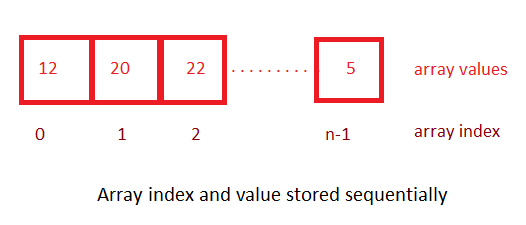# C# Array

C# arrays is a collection of same datatype, which we can access using array index ( location of element inside array). Array elements are placed into contiguous memory locations.  So, array elements can be accessed by using array name and index number.

Index of array elements start from 0 and finish at n-1, where n = number of elements in the array. Consider a integer array sample as shown below, which shows array values and index number to access it's valuesIf name of an array is `sample`, then we can get second value(22) of array as `sample`, similarly, for third value (22), we can get it like` sample`

Arrays can be 1-D array, 2-D arrays, 3-D arrays, Jagged arrays.

### Declare array in C#

Here is the syntax to declare an array in C#

``datatype arrayname[]; //we are declaring array here``

Where,

datatype = can be of any datatype like int, string, char etc, which will be stored in an array

arrayname = name of the array

Example:

``````int[] arrInt;
string[] arrStr;``````

### Define array in C#

Above, we have just declared the array, we haven't defined it, which we need to do, in order to use it.

Basically, while defining, we will be specifying the size of the array element.

Syntax

``arrayname = new datatype[size];``

arrayname = name of the array

new= keyword

datatype = type of data which will be stored in array

size = size of the array

Considering above example

``arrInt= new int;``

In the above sample, we are storing `int` array and array has can store upto 5 elements.

But, we can define and declare array in same one line as below

``datatype[] arrayname = new datatype[size];``

Example

``int[] myArr = new int; //int array with 5 elements size``

### Initialize array in C#

We have defined and decalred array until now, but haven't initialized it, we can initiliaze array by placing curly braces and placing values inside it, seperated by comma.

Example

``````int[] myArr= {10,5,6,7,8}; // int type arra initialized
string[] strArr= {"Mouse","Cat","Dog"}; //string array initialized``````

We can also declare and initialize array like this

``````int[ ] arr1 = new int[] { 1, 2, 3, 4, 5};
int[ ] arr2 = new int { 1, 2, 3, 4, 5};``````

### Example of C# Array

In this example, we will create a program to declare, initialize, and print an int array in C#

``````using System;

public class ArrayInCsharpProgram
{
public static void Main()
{
int[] arrInt= new int[] {1,2,3,4,5};
int i;
Console.WriteLine("Elements in the array are:");
for ( i = 0; i < 5; i++ )
{
Console.WriteLine(arrInt[i]);
}

}
}``````

Output

``````Elements in the array are:
1
2
3
4
5``````

### String array in C#

``````using System;

public class Program
{
public static void Main()
{
string[] names = {"John","Sam","Mick","jack"};
Console.WriteLine("names : "+names);
Console.WriteLine("names : "+names);

}
}``````

Output:

``````names : Sam
names : jack``````

In the above example, we are using string array to declare and initialize, then we are accessing array values using it's index location.

### Looping array using ForEach loop in C#

``````using System;

public class ForEachCsharpProgram
{
public static void Main()
{
string[] names = {"John","Sam","Mick","jack"};
Console.WriteLine("Elements in the array are:");
foreach (string str in names)
{
Console.WriteLine(str);
}

}
}``````

Output:

``````Elements in the array are:
John
Sam
Mick
jack``````

Foreach loop is an easy way to loop array or collections in C#. Foreach loop syntax

``````foreach (datatype element in iterable-item-like-array)
{
// body of foreach loop
}``````

Where datatype, can be any datatype like int, char, string.

We are using `in` keyword within foreach loop to iterate over the `iterable-item-like-array`. The `in` keyword selects an item from the `iterable-item-likearray `on each iteration and store it in the variable `element`, which we can use inside `foreach `loop body.